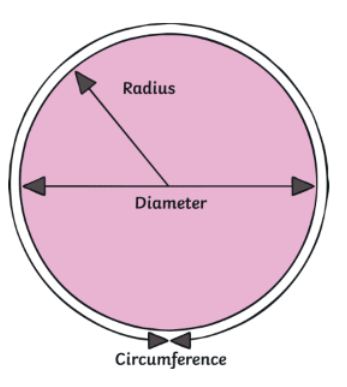Learn 5 Most Important Civil Engineering Formulas

# Learn 5 Most Important Civil Engineering FormulasCivil engineering is thought to be one of the oldest jobs in the world. From bridges to water treatment, their work helps the lives of most people in the world every single day.

A good Civil Engineer should know enough math and physics to find and solve engineering problems. If a Structural Engineer couldn’t figure out how much weight was being spread out across a bridge, that wouldn’t be a bridge we’d feel safe crossing.It is important for them to know the most important formulas used by civil engineers when they work on a construction site. Important formulas help you figure out how much material you need for shuttering work, skirting work, plastering work, painting work, cornice work, and other things.

Know more about the top important formulas a civil engineer must know!

1. Rectangle

Application:

• Shuttering Area of Rectangle column, Pile Cap, Rectangle Isolated Pad Footing etc.
• Volume of Concrete of Rectangular Column, Footing, Beam, Pile Cap, Slab etc.
• Skirting of Tile in Rectangular Column etc.Photo Courtesy: Quora

Length of Rectangle = L.

Height of Rectangle = H.

Formula

1. Perimeter or Peripheral length (P) = L+ B +L + B =2 x (L + B).
2. Area of Rectangle Cross-section = L x B.
3. Area of Rectangle (A) = Peripheral Length x Height of Rectangle.
4. Volume of Rectangle (V) = Area of Rectangle x Height of Rectangle.

2. Square

Applications:

• Square column shuttering area, Pile Cap, Footing, etc.
• Concrete Volume of Square Column, Footing, Beam, Pile Cap, Slab, and Other Structures
• Tile skirting in a square columnPhoto Courtesy: Javatpoint

Length of Square = L.

Height of Square = H.

Formula

1. Perimeter or Peripheral length of Square (P) = L + L+ L + L = 4L.
2. Area of Square Cross-section = L2.
3. Area of Square (A) = Perimeter x Height of Square = 4L x H.
4. Volume of Square (V) = Area of Square (A) x Height of Square.

3. Circle

Application:

• Calculation Shuttering Circular column area, Circular Pile area, and etc.
• Volume of Concrete of Circular Column, Circular Pile etc.
• Skirting of Tile in Circular Column etc.Photo Courtesy: Toppr

Diameter of Circle = D.

Height of Circle = H.

Important Formulas

• Perimeter (P) = 2 x π x r.
• Area of Circular Cross-section = π/4 x D2 or π r2.
• Area of Circle (A) = Peripheral Length x Height of Circle.
• Volume of Circle (V) = Area of Circle x Height of Circle.

3.1 Semi CirclePhoto Courtesy: Twinkl

Diameter of Circle = D.

Important Formulas

1. Perimeter (P) =1/2 x (Perimeter of Circle) = 1/2 x (2 x π x r).
2. Area of Semi-circle = 1/2 x (Area of Circle) = 1/2 x (π/4 x D2 ).

4. Triangle

Application:

• Calculation of shuttering area of Staircase Steps etc.
• Volume of Ramp, Staircase steps etc.Photo Courtesy: Civil Success Online

Height of Triangle =H.

Inclined Length of Triangle = C.

Length of Triangle (L) = L.

Formula

• Inclined Length (C) =√ (B2 + H2).
• Perimeter = B + H + C.
• Area of triangle cross-section (A) = 1/2 x B x H.
• Area of Triangle = Perimeter x Length of Triangle.
• Volume of Triangle (V) = Area of Triangle x Length of Triangle.

5. Frustum

Application:

• To determine the volume of concrete required for the slump test.Photo Courtesy: Pinterest

Radius of upper base = r.

Radius of Lower base = R.

Slant height = s.

Height of frustum =h.

Important Formulas

• Volume of Frustum = 1/3πh (r2 + rR + R2).
• Lateral Surface Area = π(r + R)s.
• Total surface Area = π(r +R)s + πr2 + πR2

Civil engineers also need to know how to design things and work with maps, drawings, and models, as well as how to use CAD software to make things. They need to solve potential problems before they even happen.

CITATIONS: